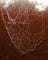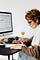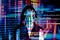# What is Dummy Variable Trap? How it can be handled using python?Photo by Pixabay from Pexels

Dummy Variable Trap occurs when predictors are multicollinear, that is one predictor can predict another.

Statistical Analysis of Dummy Variable Trap

For example, if I am having a data set like below(only 15 rows shown here), where the first 4 features are used to predict Profit. The First 3 Columns contain numerical data and the fourth column D contains categorical data. Before building a model categorical data is to be encoded.

# Linear Regression AssumptionsPhoto Credit to Karolina Grabowska from Pexels

Linear regression is used when the dataset has a linear correlation. Before building a linear regression model assumptions are to be validated. If the assumptions are violated, different methodologies must be used.

Simple linear regression has one independent variable (predictor) and a dependent variable(response) and multiple linear regression has more than one predictor to predict response.

The simple linear regression equation is represented as

## Y = β0 + β1 (X1 )+ε

Multiple linear regression equation is represented as

## Y = β0 + β1 (X1 )+β2 (X 2)+β3(X3)+β4(X4)+ε

Assumptions of Linear Regression Analysis are :

1. Linearity
2. No Heteroskedasticity
3. No omitted variable bias
4. Normality of error
5. No autocorrelation
6. No multicollinearity

1. Linearity

For linear regression analysis, there must…

# Simple Linear Regression Analysis Using PythonImage downloaded from www.piexel.com

Regression analysis is the most widely used method of prediction. Linear regression is used when the dataset has a linear correlation and as the name suggests, simple linear regression has one independent variable (predictor) and one dependent variable(response).

The simple linear regression equation is represented as y = a+bx where x is the explanatory variable, y is the dependent variable, b is coefficient and a is the intercept.

In linear regression, the best fit line will be one that will have minimum vertical distance with data points. One of the popular methods is the least square method and here the…Data Enthusiast